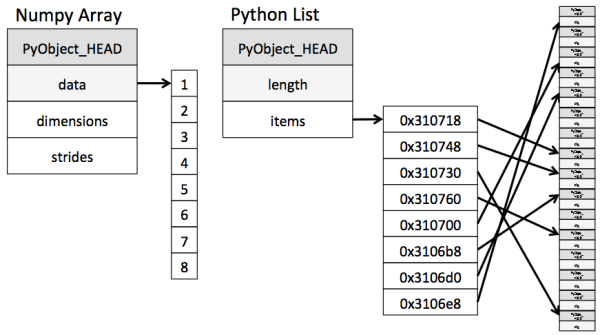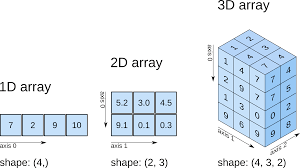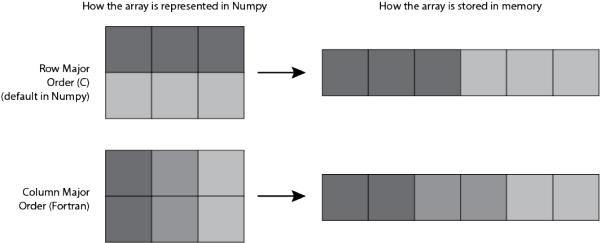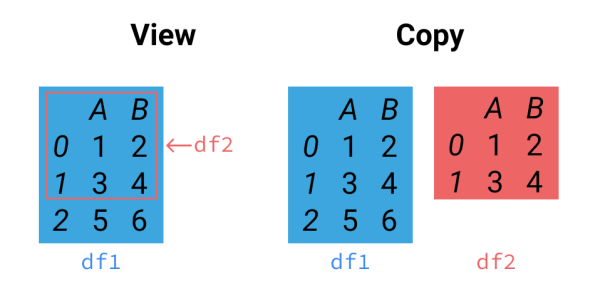# 为什么用 Numpy 还是慢, 你用对了吗?

• 我知道 Pandas 要比 Numpy 慢, 所以我尽量避免用 Pandas. 但是 Numpy (速度怪兽), 为什么还是这么慢?

## 为什么用 Numpy? ¶b=1; a=b/0.5


## 创建 Numpy Array 的结构 ¶col_major = np.zeros((10,10), order='C')    # C-type
row_major = np.zeros((10,10), order='F')    # Fortran


## 在 Axis 上的动作 ¶

a = np.zeros((200, 200), order='C')
b = np.zeros((200, 200), order='F')
N = 9999

def f1(a):
for _ in range(N):
np.concatenate((a, a), axis=0)

def f2(b):
for _ in range(N):
np.concatenate((b, b), axis=0)

t0 = time.time()
f1(a)
t1 = time.time()
f2(b)
t2 = time.time()

print((t1-t0)/N)     # 0.000040
print((t2-t1)/N)     # 0.000070


np.vstack((a,a))                # 0.000063
np.concatenate((a,a), axis=0)   # 0.000040


indices = np.random.randint(0, 100, size=10, dtype=np.int32)
a[indices, :]     # 0.000003
a[:, indices]     # 0.000006


## copy慢 view快 ¶a = np.arange(1, 7).reshape((3,2))
a_view = a[:2]
a_copy = a[:2].copy()

a_copy[1,1] = 0
print(a)
"""
[[1 2]
[3 4]
[5 6]]
"""

a_view[1,1] = 0
print(a)
"""
[[1 2]
[3 0]
[5 6]]
"""


a = np.zeros((1000, 1000))
b = np.zeros((1000, 1000))
N = 9999

def f1(a):
for _ in range(N):
a *= 2           # same as a[:] *= 2

def f2(b):
for _ in range(N):
b = 2*b

print('%f' % ((t1-t0)/N))     # f1: 0.000837
print('%f' % ((t2-t1)/N))     # f2: 0.001346


def f1(a):
for _ in range(N):
a.flatten()

def f2(b):
for _ in range(N):
b.ravel()

print('%f' % ((t1-t0)/N))    # 0.001059
print('%f' % ((t2-t1)/N))    # 0.000000


## 选择数据 ¶

a_view1 = a[1:2, 3:6]    # 切片 slice
a_view2 = a[:100]        # 同上
a_view3 = a[::2]         # 跳步
a_view4 = a.ravel()      # 上面提到了
...                      # 我只能想到这些, 如果还有请大家在评论里提出


a_copy1 = a[[1,4,6], [2,4,6]]   # 用 index 选
a_copy2 = a[[True, True], [False, True]]  # 用 mask
a_copy3 = a[[1,2], :]        # 虽然 1,2 的确连在一起了, 但是他们确实是 copy
a_copy4 = a[a[1,:] != 0, :]  # fancy indexing
a_copy5 = a[np.isnan(a), :]  # fancy indexing
...                          # 我只能想到这些, 如果还有请大家在评论里提出


Numpy 给了我们很多很自由的方式选择数据, 这些虽然都很方便, 但是如果你可以尽量避免这些操作, 你的速度可以飞起来.

1.使用 np.take(), 替代用 index 选数据的方法.

a = np.random.rand(1000000, 10)
N = 99
indices = np.random.randint(0, 1000000, size=10000)

def f1(a):
for _ in range(N):
_ = np.take(a, indices, axis=0)

def f2(b):
for _ in range(N):
_ = b[indices]

print('%f' % ((t1-t0)/N))    # 0.000393
print('%f' % ((t2-t1)/N))    # 0.000569


2.使用 np.compress(), 替代用 mask 选数据的方法.

mask = a[:, 0] < 0.5
def f1(a):
for _ in range(N):
_ = np.compress(mask, a, axis=0)

def f2(b):
for _ in range(N):

print('%f' % ((t1-t0)/N))    # 0.028109
print('%f' % ((t2-t1)/N))    # 0.031013


## 非常有用的 out 参数 ¶

a = a + 1         # 0.035230
a = np.add(a, 1)  # 0.032738


a += 1                 # 0.011219
np.add(a, 1, out=a)    # 0.008843


## 给数据一个名字 ¶

a = np.zeros(3, dtype=[('foo', np.int32), ('bar', np.float16)])
b = pd.DataFrame(np.zeros((3, 2), dtype=np.int32), columns=['foo', 'bar'])
b['bar'] = b['bar'].astype(np.float16)

"""
# a
array([(0,  0.), (0,  0.), (0,  0.)],
dtype=[('foo', '<i4'), ('bar', '<f2')])

# b
foo  bar
0    0  0.0
1    0  0.0
2    0  0.0
"""

def f1(a):
for _ in range(N):
a['bar'] *= a['foo']

def f2(b):
for _ in range(N):
b['bar'] *= b['foo']

print('%f' % ((t1-t0)/N))    # 0.000003
print('%f' % ((t2-t1)/N))    # 0.000508